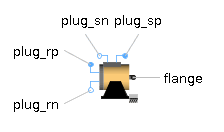Body Radiation - MapleSim Help

Lumped thermal element for radiation heat transferDescription

The Body Radiation component models the thermal radiation emitted between two bodies as a result of their temperatures. The following constitutive equation is used: ${Q}_{\mathrm{flow}}={G}_{r}\mathrm{\sigma }\left({T}_{a}^{4}-{T}_{b}^{4}\right)$, where ${G}_{r}$ is the radiation conductance and $\mathrm{\sigma }$ is the Stefan-Boltzmann constant.Computation of ${G}_{r}$

In simple cases ${G}_{r}$ may be analytically computed. The analytical equations use $\mathrm{ϵ}$, the emissivity of a body, which varies from zero if the body reflects and does not absorb radiation to one if the body absorbs all radiation (black body), The following table gives the approximate values of $\mathrm{ϵ}$ for various materials.

 Material Emissivity $\left(\mathrm{ϵ}\right)$ aluminium, polished 0.04 copper, polished 0.04 gold, polished 0.02 paper 0.09 rubber 0.95 silver, polished 0.02 wood 0.85..0.9Small convex object in a large enclosure ${G}_{r}=\mathrm{ϵ}A$ where $\mathrm{ϵ}$: Emission value of object (0..1) $A$:  Surface area of object where radiation heat transfer takes placeTwo parallel plates ${G}_{r}=\frac{A}{\frac{1}{{\mathrm{ϵ}}_{1}}+\frac{1}{{\mathrm{ϵ}}_{2}}-1}$ where ${\mathrm{ϵ}}_{1}$: Emission value of plate 1 (0..1) ${\mathrm{ϵ}}_{2}$: Emission value of plate 2 (0..1) $A$ : Area of plate1 (= area of plate2)Concentric cylinders (radiation from inner to outer cylinder) ${G}_{r}=\frac{2\mathrm{\pi }{r}_{1}L}{\frac{1}{{\mathrm{ϵ}}_{1}}+\left(\frac{1}{{\mathrm{ϵ}}_{2}}-1\right)\left(\frac{{r}_{1}}{{r}_{2}}\right)}$ where ${r}_{1}$: Radius of inner cylinder ${r}_{2}$: Radius of outer cylinder $L$: Length of the two cylinders ${\mathrm{ϵ}}_{1}$: Emission value of inner cylinder (0..1) ${\mathrm{ϵ}}_{2}$: Emission value of outer cylinder (0..1)Equations ${Q}_{\mathrm{flow}}={Q}_{{\mathrm{flow}}_{a}}+{Q}_{{\mathrm{flow}}_{b}}={G}_{r}\mathrm{\sigma }\left({T}_{a}^{4}-{T}_{b}^{4}\right)$ $\Delta T={T}_{a}-{T}_{b}$ $\mathrm{\sigma }\mathrm{is the Stefan-Boltzmann constant}$Variables

 Name Units Description Modelica ID ${Q}_{\mathrm{flow}}$ $W$ Heat flow rate from port_a -> port_b Q_flow $\Delta T$ $K$ Temperature difference between ports dTConnections

 Name Description Modelica ID ${\mathrm{port}}_{a}$ port_a ${\mathrm{port}}_{b}$ port_bParameters

 Name Default Units Description Modelica ID ${G}_{r}$ ${m}^{2}$ Net radiation conductance between two surfaces; emissivity times surface area GrModelica Standard Library The component described in this topic is from the Modelica Standard Library. To view the original documentation, which includes author and copyright information, click here.